# Alligation or Mixtures - Aptitude test, questions, shortcuts, solved example videos

Video on Alligation or Mixture - shortcuts, tips and tricks

## Alligation and Mixtures

Important terms:

1) Alligation: Rule of alligation enables us to find the ratio in which two or more ingredients at a given price must be mixed to produce a resultant mixture of desired price.

2) Mean Price: It is the cost of unit quantity of a mixture.

3) Mixture: Mixture is formed by mixing two or more quantities. It can be expressed in the form of percentage or ratio.

- 10 % of sugar in water
- A solution of water and sugar is 12 : 20, which means water : sugar = 12 : 20

According to the rule of alligation: When two ingredients are mixed,

 Cheaper Quantity (X) = (C.P. of Y) – (Mean Price) (Quantity of Y) (Mean Price) – (C.P. of X)

If a vessel contains A liters of milk and if B litres of milk is withdrawn and replaced by water, and again if B litres of mixture is withdrawn and replaced by water and this operation is replaced n times in all, then

 (Quantity of milk left after nth operation) =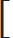(A – B)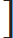n (Initial quantity of milk) A
 Quantity of milk left after nth operation = A x [A(1 – (B/A))n] A
Simplified formula to calculate quantity of milk left after nth operation = [A(1 – (B/A))n]

Tricks and Tips:

1) Rule of alligation: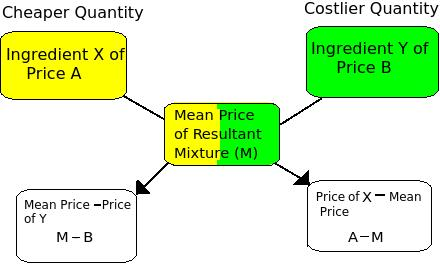Ratio = (M – B) = (B – M) (A – M) (M – A)

Points to Remember While Using the Rule of Alligation

- The three values alligated should always represent the same variable and should have same units.
- alligation of 3 values of cost gives the ratio in terms of number and vice-versa.
- If two values of cost price and selling price of the mixture are given, then in such cases first calculate the cost price of the mixture and then allegate the 3 values of cost price.
- A and B represent concentration if the numerical is based on mixing of solutions.

Question Variety

Type : 1 Based on Rule of Alligation

Examples:

Q 1. In what ratio must wheat A at Rs. 10.50 per kg be mixed with wheat B at Rs. 12.30 per kg, so that the mixture be worth of Rs. 11 per kg?

a. 13 : 5
b. 18 : 3
c. 17 : 5
d. 11 : 5
View solution

Correct option :(a)

Convert Rs into paise, to make the calculation easy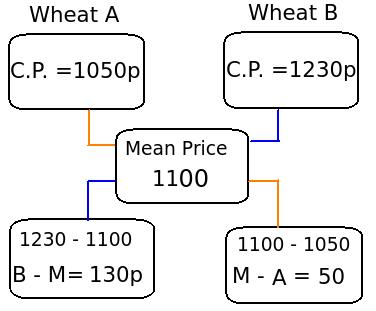Ratio = (B – M) (M – A)

The required ratio = 130 : 50 = 13 : 5

Q 2. In what ratio must a shopkeeper mix Peas and Soybean of Rs. 16 and Rs. 25 per kg respectively, so as to obtain a mixture of Rs. 19.50 ?

a. 9 : 5
b. 7 : 5
c. 11 : 7
d. 12 : 8
View solution

Correct option: (c)

Use rule of alligation, to determine the ratio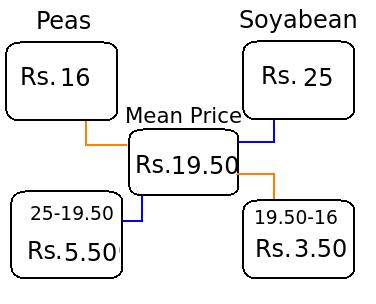The required ratio of Soybean and Peas = 5.50 : 3.50 = 11 : 7

Type 2: Calculate quantity of milk/alcohol left after nth operation = [A(1 – (B/A))n]

Examples:

Q 3. 10 gallons are drawn from a container full of alcohol and filled with water again. 10 gallons of mixture are again drawn and the container is filled with water again. If the ratio of alcohol and water left in the container is 49 : 32, then find how much quantity does the container hold?

a. 35 gallons
b. 45 gallons
c. 55 gallons
d. 60 gallons
View solution

Correct option : (b)

Initially the container contains only wine. 10 gallons of alcohol was removed and same quantity of water was added.
This process is again repeated by replacing the mixture( alcohol + water) of 10 gallons with same quantity of water. Hence, the initial quantity of wine and the final quantity of water and alcohol is the same.
1) First assume that the initial quantity of alcohol is 'A' .
2) We are given that, the ratio of alcohol and water is 49 : 32
3) Assume initial quantity of alcohol in the container = 49 + 32 = 81 ----- (This is because we have assumed that initial quantity of alcohol = final quantity of water and alcohol)
4) Subtract the quantity of alcohol replaced by water from the initial quantity of alcohol (A – B). As this operation is repeated n times, therefore (A – B)n

Therefore,

 (Quantity of alcohol left after nth operation) =(A – B)n(Initial quantity of alcohol) or (Volume of flask) A
 49 = (A – 10)2 81 A

Solving, we can find the value of A (initial quantity of alcohol)
A = 45 gallons

Q 4. A container is filled with a mixture of water and milk in the ratio of 3 : 5. Find the quantity of mixture to be drawn off and replaced with water, in order to get the mixture as half milk and half water.

a. 2 : 3
b. 1 : 1
c. 1 : 5
d. 1 : 4
View solution

Correct option : (c)

A container contains milk and water in the ratio of 3 : 5. This means that the vessel contains 8 litres of mixture.

Assume that x litres of this mixture is replaced with water.

From the mixture containing water and milk of 3 : 5, x quantity of mixture is withdrawn and is replaced by water of the same quantity in the mixture. From the mixture (3x)/(8) part of water is removed and x quantity of water is added.

 1) Quantity of water in the newly formed mixture = [3 – ( 3x ) + x] 8
---- (3 is the quantity of water, x is the quantity of mixture replaced by water)
 2) Similarly, quantity of milk in the newly formed mixture = [5 – ( 5x )] 8
---- (Here x is not added because only water was added in the mixture and not the syrup)

Therefore,
 [ 3 – ( 3x ) + x] = [5 – ( 5x )] 8 8

Solving 1 and 2, we get

5x + 24 = 40 -5x

x = 8 / 5
 So the part of mixture replaced from 8 litres = 8 x 1 = 1 5 8 5

Type 3: Given : Profit and Loss obtained,
1) When S.P. = C.P.
2) Find quantity added to gain profit

Examples:

Q 5. Find in what ratio must water be mixed with alcohol to gain 10% profit by selling the mixture at cost price.

a. 1 : 5
b. 1 : 10
c. 1 : 15
d. 1 : 20
View solution

Correct option : (b)

Assume, C.P. and S.P. of alcohol = Re. 1 per litre

 The general formula to calculate C.P. in case of profit = 100 x S.P. (100 + Gain%)

Here, 10 % profit is gained, therefore
 Cost price of 1 litre of mixture = Rs. 100 x 1 = Rs. 100 = 10 (100 + 10) 110 11

Rs. 10 / 11 is the cost price of mixture.

Now, use the rule of alligation to determine the ratio of water and alcohol.

Water is free of cost, hence C.P. of water is zero.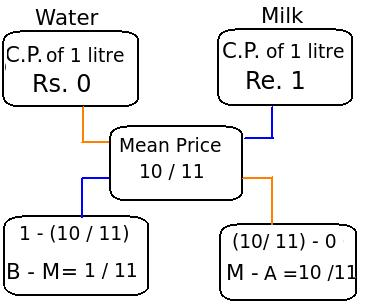The ratio of milk and water = 1 : 10 = 1 : 10 11 11

Q 6. A shopkeeper has 100 kg of salt. He sells part of the total quantity A at 7% profit and the rest B at 17 % profit. If he gains 10 % profit on the whole quantity, then find how much is sold at 7 % profit?

a. 30 kg
b. 35 kg
c. 40 kg
d. 45 kg
View solution

Correct option : (a)

Assume that A and B are two parts of the mixture. To determine the quantity A and B, first calculate ratio of A : B.

Given:
1) Selling price of mixture with 10% profit = Rs. 110
2) With 17 % profit, the selling price of A = Rs. 117
3) With 7 % profit, the selling price of B = Rs. 107

Now, this question can be easily solved by using the rule of alligation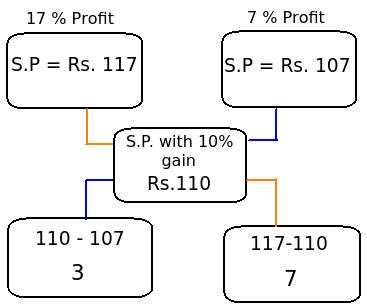Now, the ratio of A : B = 3 : 7

Let the quantity of part A be 3x and part B be 7x in the total quantity of 100 kg.

Therefore, 3x + 7x = 100
10x = 100
x = 10

Quantity of part A = 3x = 3 x 10 = 30 kg
Quantity of part B = 7x = 7 x 10 = 70 kg

Type 4 : If three types of mixtures are given. Find
1) Price of third variety when first two mixtures are mixed.
2) Ratio of quantities in newly formed mixture.

Examples:

Q 7. Sugar A worth Rs. 130/kg and B of Rs. 120/kg are mixed with a third variety C in the ratio of 1 : 1 : 2. If the mixture is worth Rs. 160, then find the price of third variety of sugar.

a. Rs. 195
b. Rs. 200
c. Rs. 225
d. Rs. 230
View solution

Correct option: (a)

 1) First calculate the average of A and B variety of sugar: (120 + 130) = Rs. 125 2
2) Now, the mixture is formed by two varieties of sugar, one at Rs. 125 /kg and assume the cost of type C Rs. x. It is formed in the ratio of 2 : 2, i.e 1 : 1

Use the rule of alligation, to easily determine the unknown quantity.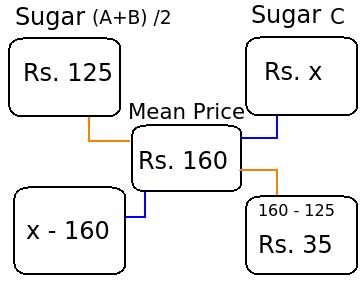Therefore,
 (x - 160) = 1 35

x = 35 + 160 = Rs. 195

The cost of third variety of sugar C = Rs. 195

Q 8. Two containers P and Q contain milk and water in the ratio of 5 : 2 and 7 : 6 respectively. Find the ratio in which these two mixtures can be mixed so that a new mixture formed in the container R is in the ratio of 8 : 5.

a. 5 : 6
b. 4 : 9
c. 7 : 9
d. 9 : 7
View solution

Correct Option:(c)

Let the cost price of milk be Re. 1 per litre.

Therefore, cost of milk in 1 litre of mixture in

 Container A (Milk : Water = 5 : 2) = 5 x Re. 1 = Re. 5 7 7
 Container B (Milk : Water = 7 : 6) = 7 x Re. 1 = Re. 7 13 13
 Container C (Milk : Water = 8 : 5) = 8 x Re. 1 = Re. 8 13 13

Now use the rule of alligation, to find the required ratio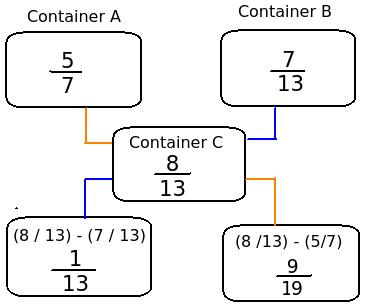The required ratio of milk and water:
 1 : 9 13 91

Simplifying, we get 7 : 9

Practice questions on Alligation or Mixture
Problems on Trains - Aptitude test, questions, shortcuts, solved example videos
Problems on Trains - Quantitative aptitude tutorial with easy tricks, tips, short cuts explaining the concepts. Online aptitude preparation material with practice question bank, examples, solutions and explanations. Video lectures to prepare quantitative aptitude for placement tests and competitive exams like MBA, Bank exams, RBI, IBPS, SSC, SBI, RRB, Railway, LIC, MAT. Very useful for freshers, engineers, software developers taking entrance exams. Learn and take practice tests!
Time and Distance - Aptitude test, questions, shortcuts, solved example videos
Time and Distance - Quantitative aptitude tutorial with easy tricks, tips, short cuts explaining the concepts. Online aptitude preparation material with practice question bank, examples, solutions and explanations. Video lectures to prepare quantitative aptitude for placement tests and competitive exams like MBA, Bank exams, RBI, IBPS, SSC, SBI, RRB, Railway, LIC, MAT. Very useful for freshers, engineers, software developers taking entrance exams. Learn and take practice tests!
Time and Work - Aptitude test, questions, shortcuts, solved example videos
Time and Work - Quantitative aptitude tutorial with easy tricks, tips, short cuts explaining the concepts. Online aptitude preparation material with practice question bank, examples, solutions and explanations. Video lectures to prepare quantitative aptitude for placement tests and competitive exams like MBA, Bank exams, RBI, IBPS, SSC, SBI, RRB, Railway, LIC, MAT. Very useful for freshers, engineers, software developers taking entrance exams. Learn and take practice tests!